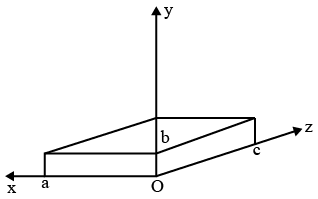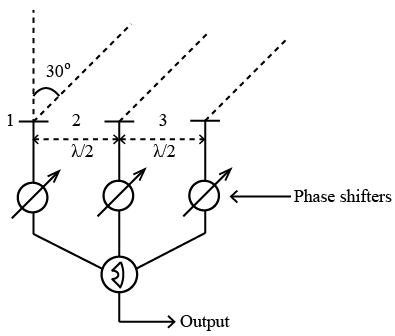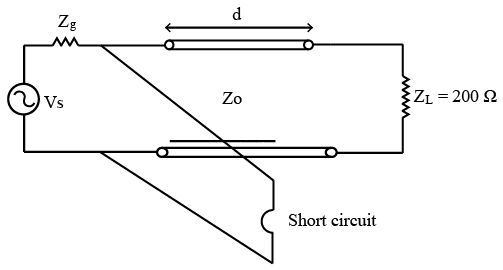# ISRO Scientist or Engineer Electronics 2020

Instructions

For the following questions answer them individually

Question 1

# The plane wave propagating through the di-electric has the magnetic field component as $$H=20 e^{-ax} \cos (\omega t-0.25) a_{y} \frac{A}{m}$$ $$(a_{x},a_{y},a_{z}$$, are the unit vectors along x, y and z axis respectively ) Determine the Polarization of the waveQuestion 2

# In Free space, $$H=0.1 cos(\omega t-\beta x) a_{z} \frac{A}{m}$$  ($$a_{x}, a_{y}, a_{z}$$ ,are the unit vectors along x, y and z axis respectively ) The Total power passing through a square plate of side)10 cm on plane $$x + 2y = 1.0$$, is approximately :Question 3

# Consider the rectangular cavity as shown below :If $$a = c > b$$, The Dominant mode of resonance corresponding to the above Rectangular cavity isQuestion 4

# A video camera generates data at a rate of 5 Mbps. The data is channel coded at rate $$\frac{1}{3}$$ and 8 PSK modulated. Which of the following statements is correct?Question 5

# Evaluate $$\int_{-\infty}^{\infty} x^{4} f(x) dx$$, where $$f(x)=\frac{1}{\sqrt{2 \pi}}e^{-\left(\frac{x^{2}}{2}\right)}, x \in(-\infty,\infty)$$Question 6

# Consider a transformation $$T:R^{3} \rightarrow R^{2}$$ where $$R^{3}$$ and $$R^{2}$$ represent three and two dimensional real column vectors respectively. Also, T(x) = Ax for some matrix A and for each x in $$R^{3}$$ How many rows and colums does A have and what is its maximum possible rank?Question 7

# The output of a three element co-linear antenna array operating in a free space environment is combined after appropriate phase shifting) to maximize the signal received from a particular direction as shown in figureIf the inter-element spacing is half of the signal wavelength and the direction of maximum response is $$30^\circ$$ from line perpendicular to the array ,what are the phases to be apllied to the each element? Consider the first element as the reference?Question 8

# The transmission line of charecteristic impedance 50 Ω and feeding a purely resistive load of 200 Ω uses single quarter wavelength long short circuit stub which is placed at a distance d from the load .The VSWR on the transmission line section of length d and VSWR on the stub respectively areQuestion 9

# The plane Y =O carries a uniform current density of -20 k $$\frac{mA}{m}$$ The magnetic field intensity at X =1,Y =10 and Z = —2 isQuestion 10

# A Charge of 2 Coulombs is placed near a grounded conducting plate at a distance of m. The force acting between the charge of 2 C and ground conducting plate in Newton isOR